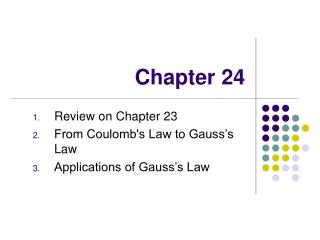# Chapter 24 - PowerPoint PPT PresentationDownload PresentationChapter 24

Chapter 24Download Presentation## Chapter 24

- - - - - - - - - - - - - - - - - - - - - - - - - - - E N D - - - - - - - - - - - - - - - - - - - - - - - - - - -
##### Presentation Transcript

1. Chapter 24 Review on Chapter 23 From Coulomb's Law to Gauss’s Law Applications of Gauss’s Law

2. Review on Chapter 23: Coulomb’s Law and the electric field definition • Coulomb’s Law: the force between two point charges • The electric field is defined as and is represented through field lines. • The force a charge experiences in an electric field

3. Two examples Example 23.9 (page 662) Example 23.10 (page 663)

4. From Coulomb’s Law to Gauss’s law Electric Flux through a perpendicular plane • Electric flux is the product of the magnitude of the electric field and the surface area, A, perpendicular to the electric field: ΦE = EA • Compare to a water flux in a tube: • ΦW = –V1A1= V2A2 This sign means water flows into the tube, by convention.

5. Electric Flux, plane with an angle θ • When the field lines make an angle θwith the direction (i.e., the normal) of the surface, the flux is calculated as: This expression is valid for electric field E that is constant all over the area A. Note: `direction’ of a surface area vector A is defined as the (outwards) normal to that surface.

6. Electric Flux, General • In the more general case, look at a small area element • In general, this becomes • The surface integral means the integral must be evaluated over the surface in question • In general, the value of the flux will depend both on the field pattern and on the surface • When the surface is closed, the direction of the surface (i.e. the normal to it) points outwards. • The unit of electric flux is N.m2/C

7. Example 1: flux through a cube of a uniform electric field • The field lines pass through two surfaces perpendicularly and are parallel to the other four surfaces • For side 1, ΦE = -El 2 • For side 2, ΦE = El 2 • For the other sides, ΦE = 0 • Therefore, Φtotal = 0

8. Example 2: flux through a sphere with a charge at its center. From Coulomb’s Law to Gauss’s Law • A positive point charge, q, is located at the center of a sphere of radius r • According to Coulomb’s Law, the magnitude of the electric field everywhere on the surface of the sphere is • The field lines are directed radially outwards and are perpendicular to the surface at every point, so • Combine these two equations, we have

9. Gauss’s Law • Closed surfaces of various shapes (Gaussian surfaces) can surround the charge • Only S1 is spherical • The flux through all other surfaces (S2 and S3) are the same. • These surfaces are all called the Gaussian Surface. • Gauss’s Law (Karl Friedrich Gauss, 1777 – 1855): • The net flux through any closed surface surrounding a charge q is given by q/εo and is independent of the shape of that surface • The net electric flux through a closed surface that surrounds no charge is zero • Since the electric field due to many charges is the vector sum of the electric fields produced by the individual charges, the flux through any closed surface can be expressed as • Gauss’s Law connects electric field with its source charge

10. Gauss’s Law – Summary • Gauss’s law states • qin is the net charge inside the Gaussian surface • represents the electric field at any point on the surface • is the total electric field at a point in space and may have contributions from charges both inside and outside of the surface • Although Gauss’s law can, in theory, be solved to find for any charge configuration, in practice it is limited to a few symmetric situations